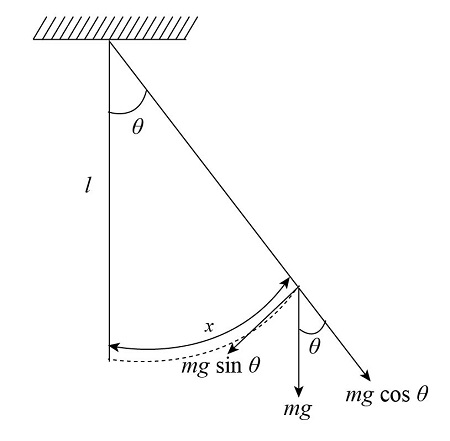# (a) Pendulum 2 has a string twice as long as pendulum 1. If we define x as the distance traveled...

## Question:

(a) Pendulum 2 has a string twice as long as pendulum 1. If we define x as the distance traveled by the bob along a circle away from the bottom, how does the k of pendulum 2 compare with the k of pendulum 1?

(b) Based on the answer from (a), how does the period of pendulum 2 compare with the period of pendulum 1? Give a numerical ratio.

## Spring Constant:

Spring constant is the property of the spring, which shows that how much amount of force is required to stretching the spring by unit displacement. Spring can be made of different material which has different spring constant value.

Become a Study.com member to unlock this answer! Create your account

(a)

Free body diagram for pendulum 1 is given as below.The expression to find the angle is,

{eq}\begin{align*} \theta ...Practice Applying Spring Constant Formulas

from

Chapter 17 / Lesson 11
3.4K

In this lesson, you'll have the chance to practice using the spring constant formula. The lesson includes four problems of medium difficulty involving a variety of real-life applications.